# Lewis Structures with LaTeX VIDEO

Lewis structures are used to illustrate the valence electrons of an atom. The video below explains how to make these diagrams using LaTeX. For chemistry students this is a great intro to using LaTeX for completing assignments.

# Making Bar Graphs in LaTeX VIDEO

It seems you can do anything with LaTeX. The example in the video below will show you how to make bar graphs. This is useful if you need to share your file and do not want to have to attach a bunch jpegs in a folder when sending it.# Creating Bar Graphs in LaTex

LaTex is a highly flexible typesetting program that seems to be capable of almost anything. Here, we will learn how to make bar graphs use this language.

Simple Bar Graph

Below is the code and the bar graph for simple bar graph.

Here is what is happening in the code

1. Line 1-2 we declare our document class and load the only package we need which is pgfplots.
2. Line 3-5 we declare the various environments needed
3. Line 6-12 are all the details we need to create the axis on which our data will be.
4. Line 7 gives the title of the graph
5. Line 8 makes the graph a bar graph
6. Line 9 places the numbers above the bars in the bar graph to make it easier to read. This line also controls how thick the bars are
7. Line 10 sets the names for the x-axis
8. Line 11 makes sure each bar only has one name
9. Line 13 creates the plot. Inside the curly braces you have in parentheses the name of the group and the frquency.
10. The rest of the code closes the enviornments

Multiple Bars

In the code below we will add several bar graphs to one plot and also add a legend. The code is mostly the same below.

The new information is in lines 12-15. These lines contain the information for the legend. The at argument tells where to put the legend at, anchor tells LaTex how to hold it and the column sep determines the width of the column.

The next important information is in lines 19-23. Here is were we add additional bars. The arguments in side still tell LaTex what color the numbers above the bar should be as well as the color of the bar graphs. The coordinates is were you can hand code the values for the x axis.

The final example will use raw data already available in LaTex to make a bar graph.

Using Data

Below is the code followed by the bar plot

New information is found first in lines 4-9. Here we use a command called “pgfplotstableread” in order to create the table. The table is found in lines 5-9. The first row sets the columns of the table and lines 6-8 is our actual data. In line 9 we give our table a name called “mydata.” It is important to note that this table will not appear in your pdf. Rather this table is just for storing information.If you want to show the table you need to make a table the traditional way.

Line 15 has the names of the groups in our data which were animal names. The next major change is in line 18. Here, we use the “addplot” command to add a table with the x as animal and the y as weight. Next to this information in curly braces is the name of the dateset called mydata.

If you look at the table you can see that we have information on the animals weight and speed but the bar plot only shows weight. In Line 19 there was some code in red which means that it was not compiled. By removing the percent sign we can run all the code and get the following.

The idea of adding to a bar plot remains the same. Just create another instance of the command and run it.

Conclusion

Making bar plots in LaTex is another convenient tool. It allows you to manipulate the data inside LaTex rather then having to pull images from your folder. If your data is stable and not going to change this might be worth while.

# APA with LATEX VIDEO

APA is one of many referencing systems. In this video, we look at how to use APA within LATEX. This is a great tool for avid users of LATEX who need knowledge of applying APA within this context. Be sure to like and leave a comment  after watching the video.# Poster in LaTeX VIDEO

Making posters in LaTeX# Making Tables with LaTeX

Tables are used to display information visually for a reader. They provide structure for the text in order to guide the comprehension of the reader. In this post, we will learn how to make basic tables.Basic Table

For a beginner, the coding for developing a table is somewhat complex. Below is the code followed by the actual table. We will examine the code after you see it.

\documentclass{article}
\begin{document}
\begin{tabular}{ccc}
\hline
Vegetables & Fruits & Nuts\\
\hline
lettace & mango & almond\\
spinach & apple & cashews\\
\hline
\end{tabular}
\end{document}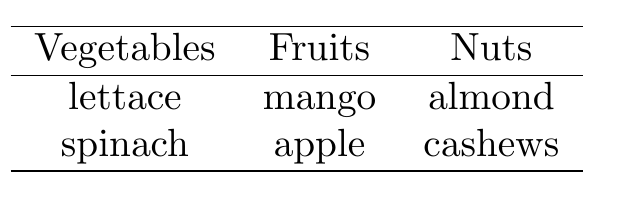We will now go through the code.

• Line 1 is the preamble and tells LaTeX that we are making an article document class.
• Line 2 is the declaration  to begin the document environment
• Line 3 is where the table begins.  We create a tabular environment. IN the second set of curly braces we used the argument “ccc” this tells LaTeX to create 3 columns and each column should center the text. IF you wan left justification to use “l” and “r” for right justification
• Line 4 uses the “\hline” declaration this draws the top horizontal line
• Line 5 includes information for the first row of the columns. The information in the columns is separated by an ampersand ( & ) at the end of this information you use a double forward slash ( \\ ) to make the next row
• Line 6 is a second “\hline” to close the header of the table
• Line 7 & 8 are additional rows of information
• Line 9 is the final “\hline” this is for the bottom of the table
• Lines 10 & 11 close the tabular environment and the document

This is an absolute basic table. We made three columns with centered text with three rows as well.

Table with Caption

A table almost always has a caption in academics. The caption describes the contents of the table. We will use the example above but we need to add several lines of code. This is described below

• We need to create a “table” environment. We will wrap this around the “tabular” environment
• We need to use the “\caption” declaration with the name of the table inside curly braces after we end the “tabular” environment but before we end the table environment.
• We will also add the “\centering” declaration near the top of the code so the caption is directly under the table

Below is the code followed by the example.

\documentclass{article}
\begin{document}
\begin{table}
\centering
\begin{tabular}{ccc}
\hline
Vegetables & Fruits & Nuts\\
\hline
lettace & mango & almond\\
spinach & apple & cashews\\
\hline
\end{tabular}
\caption{Example Table}
\end{table}
\end{document}Conclusion

We explored how to develop a basic table in LaTeX. There are many more features and variations to how to do this. This post just provides some basic ideas on how to approach this.

# Making Presentations in LaTeX VIDEO

Making presentations in LaTeX# Making Diagram Trees with LaTeX

There are times when we want to depict hierarchical relationships in a diagram. Examples include workflow chart, organizational chart, or even a family tree. In such situations, the tree diagram feature in LaTeX can meet the need.This post will provide an example of the development of a tree diagram used the tikz package. Specifically, we will make a vertical and a horizontal tree.

Vertical Tree

First I want you to see what our final product looks like before we go through each step to make it.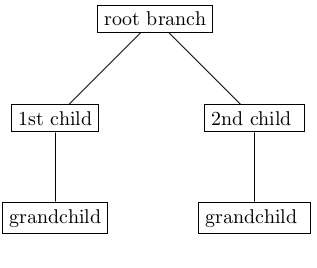As you can see it is a simple tree.

To develop the tree you need to setup the preamble with the following.

\documentclass{article}
\usepackage{tikz}
\begin{document}
\end{document}

There is nothing to see yet. All we did was set the documentclass to an article and load the tikz package which is the package we will use to make the tree.

The next step will be to make a tikzpicture environment. We also need to set some options for what we want our nodes to look like. A node is a created unit in a picture. In our completed example above there are 5 nodes or rectangles there. We have to set up how we want these nodes to look. You can set them individually or apply the same look for all nodes. We will apply the same look for all of our nodes using the every node/.style feature. Below is the initial setup for the nodes. Remeber this code goes after \begin{document} and before \end{document}

   \begin{tikzpicture}
[sibling distance=10em,level distance=6em,
every node/.style={shape=rectangle,draw,align=center}]
\end{tikpicture}

The options we set are as follows

• sibling distance = how far apart nodes on the same level are
• level distance = how far apart nodes on different adjacent levels are
• every node/.style = sets the shape and text alignment of all nodes

We are now ready to draw our tree. The first step is to draw the root branch below is the code. This code goes after the tikzpicture options but before \end{tikzpicture}.

\node{root branch};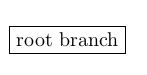We will now draw our 1st child and grandchild. This can be somewhat complicated. You have to do the following

• Remove the semicolon after {root branch}
• Press enter and type child
• make a curly brace and type node
• make another curly brace and type 1st child and close this with a second curly brace
• press enter and type child
• type node and then a curly brace
• type grandchild and close the curly braces three times
• end with a semicolon

Below is the code followed by a picture

\node{root branch}
child{node{1st child}
child{node{grandchild}}};We now repeat this process for the second child and grandson. The key to success is keeping track of the curly braces and the semicolon. A Child node is always within another node with the exception of the root. The semicolon is always at the end of the code. Below is the code for the final vertical tree.

\node{root branch}
child{node{1st child}
child{node{grandchild}}}
child{node{2nd child }
child{node{grandchild}}};Horizontal tree

Horizontal trees follow all the same steps. To make a horizontal tree you need to add the argument “grow=right” to the options inside the brackets. Doing so and you will see the following.Conclusion

As you can see, make diagram trees is not overly complicated in LaTeX. The flexibility of the tikz package is truly amazing and it seems there are no limits to what you can develop with it for visual representations.

# Insert Images into LaTeX VIDEO

Inserting images into LaTeX

# Drawing Diagrams in LaTeX

There is an old saying that most of us are familiar with that says that “a picture is worth a thousand words.” Knowing means that a communicating cannotonly include text but most also incorporate visuals as well. LaTeX allows you to develop visuals and diagrams using various packages for this purpose.

The visuals we will make are similar to those found in Microsoft Word Smart Graphics. One of the main advantages of using code to make diagrams is that they are within the document and you do not need to import images every single time you compile the document. If the image disappears it will not work but as long as the code is where you can always regenerate it.

In this post, we will use the “smartdiagram” package to make several different visuals that can be used in LaTeX. The types we will make are as follows…

• Flow diagram
• Circular diagram
• Bubble diagram
• Constellation diagram
• Priority diagram
• Descriptive diagram

The code for each individual diagram is almost the same as you will see. The preamble will only include the document class of “article” as well as the package “smartdiagram”. After this, we will create are document environment. Below is the preamble and the empty document environment.

\documentclass{article}
\usepackage{smartdiagram}
\begin{document}
\end{document}

Flow Diagram

The flow diagram is a diagram using boxes with arrows pointing from left to right in-between each box until the last box has an arrow that points back to the first bo indicating a cyclical nature. Below is  the code followed by the diagram

\smartdiagram[flow diagram:horizontal]{Step 1,Step2,Step3,Step4}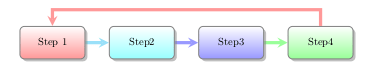The syntax is simple.

1. Call the declaration “\smartdiagram”
2. Inside the brackets, you indicate the type of diagram which was “flow diagram: horizontal” for us.
3. Next, you indicate how many boxes by typing the text and separating them by commas inside the curly braces.

This pattern holds for most of the examples in this post.

Circular Diagram

Below is a circular diagram. The syntax for the code is the same. Therefore, the code is below followed by the diagram

\smartdiagram[circular diagram:clockwise]{Step 1,Step2,Step3,Step4}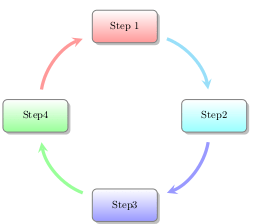Bubble Diagram

The same syntax as before. Below is the code and diagram.

\smartdiagram[bubble diagram]{Step 1,Step2,Step3,Step4}Constellation Diagram

This diagram looks similar to the bubble diagram but has arrows jutting out of the center. The syntax is mostly the same.

\smartdiagram[constellation diagram]{Step 1,Step2,Step3,Step4}Priority Descriptive Diagram

This diagram is useful if the order matters

\smartdiagram[priority descriptive diagram]{Step1, Step2, Step3,Step4}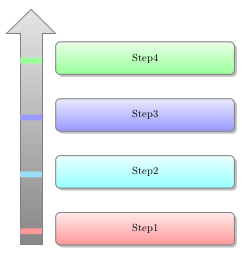Descriptive Diagram

The coding for the descriptive diagram is slightly different. Instead of one set of curly braces, you have a set of curly braces within a set of curly braces within a final set of curly braces. The outer layer wraps the entire thing. The second layer is for circles in the diagram and the inner curly braces are for adding text to the rectangle. Each double set of curly braces are separated by a comma. Below is the code followed by the diagram.

\smartdiagram[descriptive diagram]{
{Step 1,{Sample text, Sample text}},
{Step2,{More text, more text}},
{Step3,{Text again, text again}},
{Step4,{Even more text}}
}Hopefully, you can see the formatting of the code and see how everything lines up.

Conclusion

Developing diagrams for instructional purposes is common in many forms of writing. Here, we simply look at creating diagrams using LaTeX. The power of this software is the ability to create almost whatever you need for communication.

# Making Tables in LaTeX VIDEO

Making tables in LaTeX# Making Presentations in LaTeX

One of the more interesting abilities of LaTeX is the ability to make presentations similar to those that are commonly made with PowerPoint. In this post, we will explore this capability of generating presentations with LaTeXSetting Up The Preamble

The document class used for making presentations is called “beamer”. With this, you also need to set the theme of the presentation. The theme is the equivalent of a template in powerpoint. For our purposes, we will use the Singapore theme. After doing this the preamble is complete for our example

\documentclass{beamer}
\usetheme{Singapore}

Title Page

We will create the title page of the document. This involves using the “frame” environment. The code is below followed by the actual example.

\documentclass{beamer}
\usetheme{Singapore}
\begin{document}
\title{Example Project}
\subtitle{For Blog}
\author{Yours Truly}
\date{June 20, 2099}
\begin{frame}
\titlepage
\end{frame}
\end{document}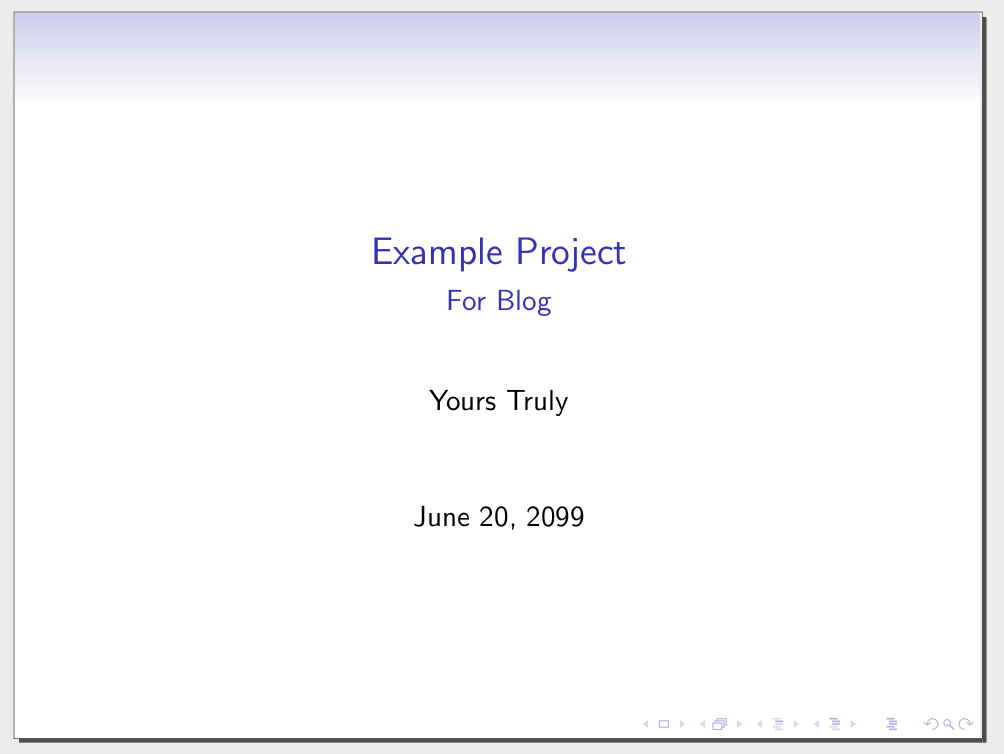Overview of Presentation

Another section that you can include is a table of contents. This will allow you to provide a big picture of what to expect in the presentation. The beamer class does not include animation so to make bullets appear LaTeX will create several slides and each slide will include one additional piece of information. This gives the appearance of animation when in fact it is a new slide. Below is the code with the additional information in bold. Unfortunately, you will not be able to see anything after this step because our example is incomplete.

\documentclass{beamer}
\usetheme{Singapore}
\begin{document}
\title{Example Project}
\subtitle{For Blog}
\author{Yours Truly}
\date{June 20, 2099}
\begin{frame}
\titlepage
\end{frame}
\begin{frame}{Outline}
\tableofcontents[pausesections]
\end{frame}
\section{Beginning}
\section{Middle}
\section{End}

Completed Presentation

We will now make several different slides that have some sample text. On each slide, we will use an “itemize” environment in order to create bullets. The bullets help to organize the text visually.  Below is the final code followed by several pictures of what it should look like.

\documentclass{beamer}
\usetheme{Singapore}
\begin{document}
\title{Example Project}
\subtitle{For Blog}
\author{Yours Truly}
\date{June 20, 2099}
\begin{frame}
\titlepage
\end{frame}
\begin{frame}{Outline}
\tableofcontents[pausesections]
\end{frame}
\section{Beginning}
\section{Middle}
\section{End}
\begin{frame}{Beginning}
\begin{itemize}
\item first point
\item Second point
\end{itemize}
\end{frame}
\begin{frame}{Middle}
\begin{itemize}
\item first point
\item Second point
\end{itemize}
\end{frame}
\begin{frame}{End}
\begin{itemize}
\item first point
\item Second point
\end{itemize}
\end{frame}
\end{document}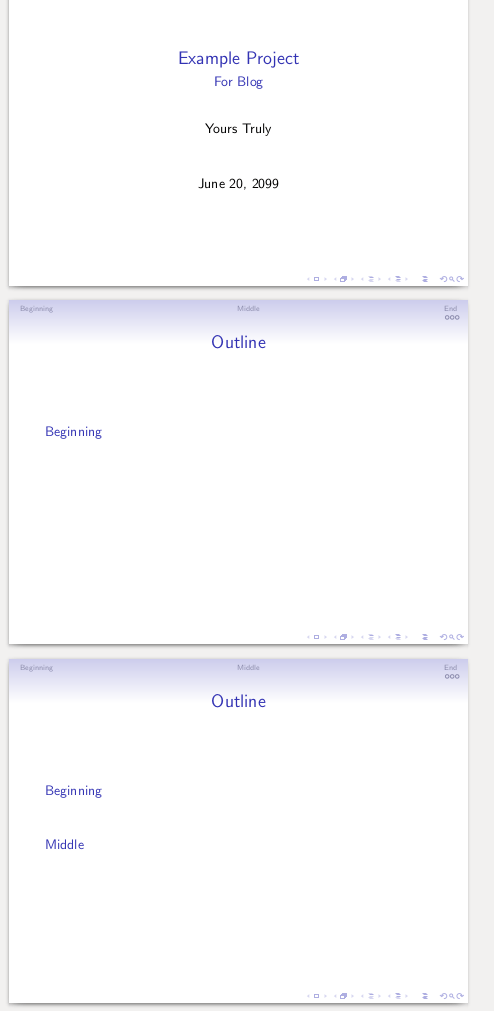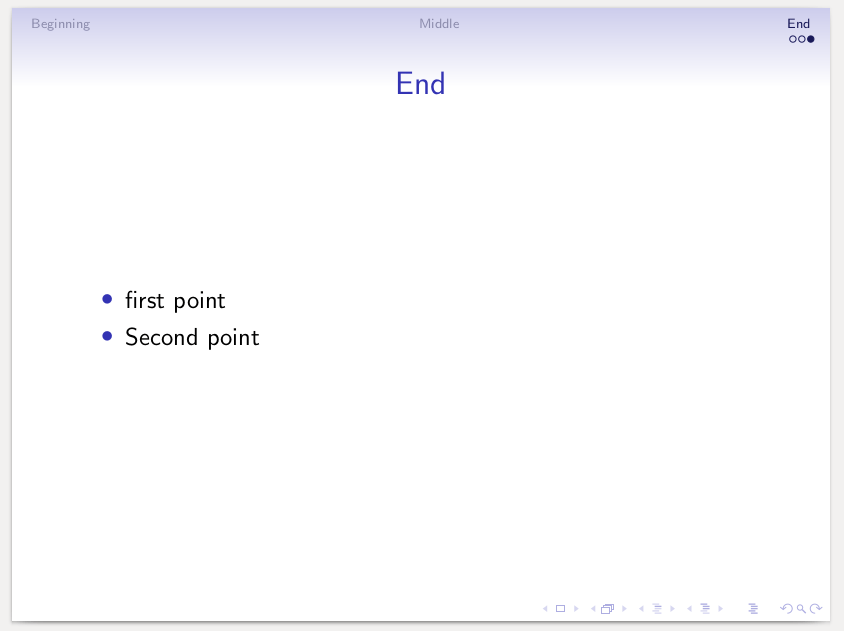Conclusion

The beamer class allows a person to develop simple efficient presentations using LaTeX. The main advantage may be speed. As you can type and add content fast simply with a few keystrokes rather than with mouse clicks. However, many people would find this cumbersome and you can do a great deal of typing using the outline view in Powerpoint. Despite this, it is good to know that LaTeX provides this feature.# Designing a Poster in LaTeX

LaTeX provides the option of being able to make posters. This can be useful for academics who often present posters at conferences in order to share their research. In this post, we will go through the development of a poster using LaTeX.First, we will setup the preamble and title of the poster. The document class is “tikzposter” with a1paper and size 25pt font. We will use the “graphicx” package for inserting images, the “lipsum” package for dummy text, and the “multicol” package to divide the poster into columns. The theme we are using is “Rays” and is one of many available themes.

Before we begin the coding it will be beneficial if you see what the final product looks like.This poster has two columns and a block along the bottom. The column 1 to the left has two blocks A and B. Block A has an inner block. In column 2, we have a block with a picture in it.

Coding

Next, we begin the document and insert the code for the title. Below is the code followed by what are document look likes so far.

\documentclass[25pt,a1paper]{tikzposter} %size is 84cmX120cm
\usepackage{graphicx}
\usetheme{Rays} %theme of poster design
\usepackage{lipsum} %for dummy text
\usepackage{multicol}
\begin{document}
\title{This is Amazing}
\author{ERT Blogger}
\maketitle
\end{document}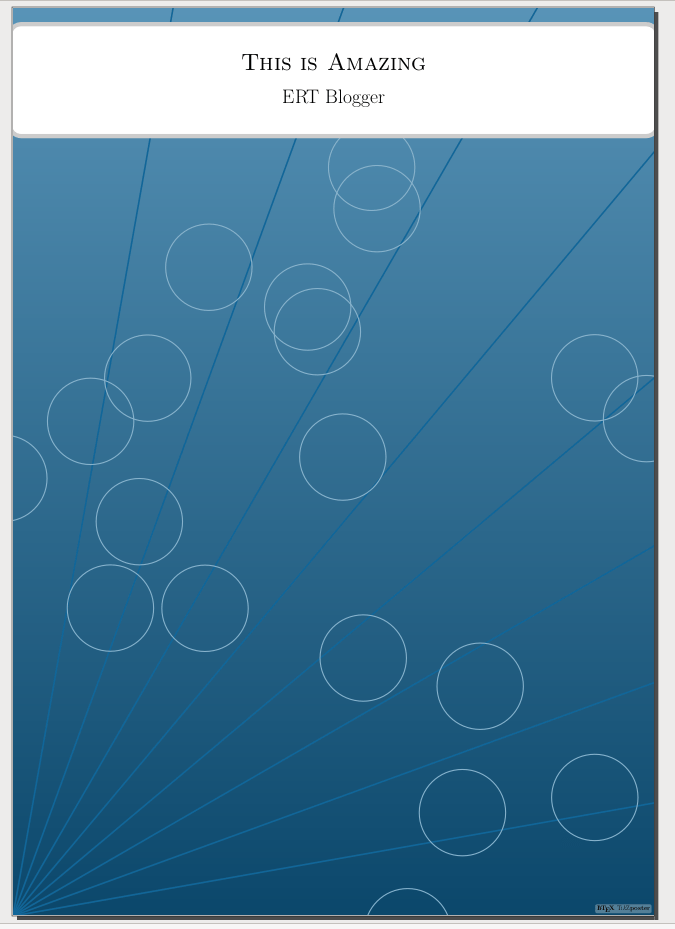Creating Columns

How you design the poster is up to you but in our example, we are going to create two columns with a horizontal block across the bottom. Column one will use 65% of the available space while column 2 will use the remaining 35%. We will not include the code for the horizontal block along the bottom yet.  For now, just look at the code and after the next step, you can copy it if you want.

\begin{columns}
%COLUMN 1
\column{.65}%use 65% of the available space for this column
%COLUMN 2
\column{.35} %last 1/3 of space
\end{columns}

Making Blocks

Inside column one, we are going to place two blocks of text called Block A and Block B. Inside each block you can design it however you want. You can even have blocks within blocks.

For Block A we will make a title, a small bit of text, a colored box with some bullets, more text, and lastly an inner-block with some math text. The \bigskip declaration provides spacing and the \lipsum declaration provides dummy text. The code is below followed by a screenshot of the current poster. This code must be placed before the \end{column} command.

\begin{columns}
%COLUMN 1
\column{.65}%use 65% of the available space for this column
%Block A
\block{More Examples of LaTeX}{
\bigskip
You can even put stuff in colored boxes.\\
\coloredbox{\begin{itemize}
\item Point 1
\item point 2
\end{itemize}}
\lipsum
\bigskip
\innerblock{here is some math for fun}
{\begin{center}
$2^5+5x-\frac{2}{x} * 3= y$
\end{center}
}
}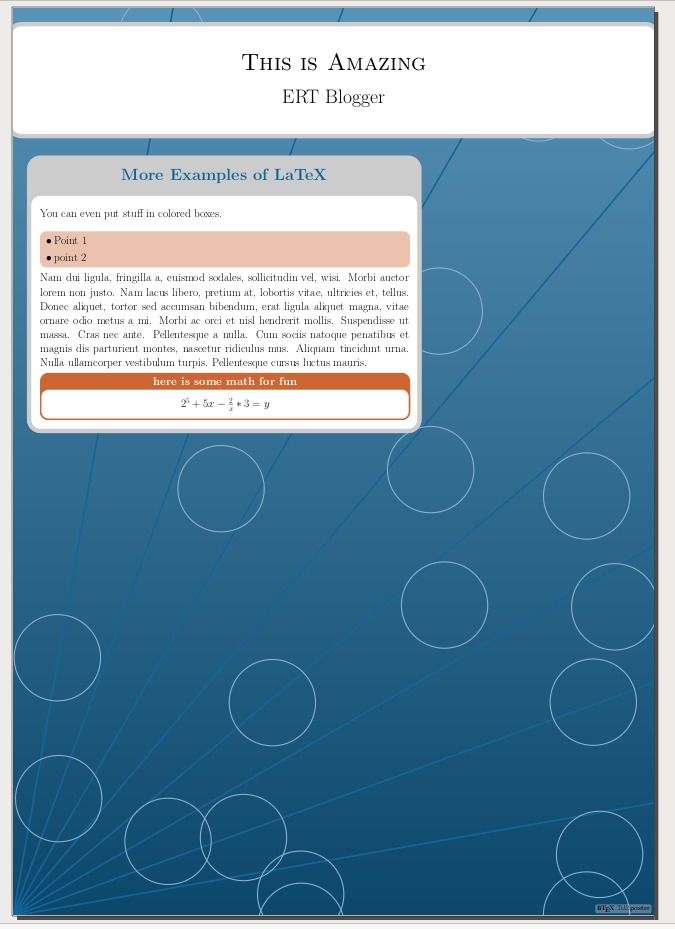Block B is much simpler and includes a title with some dummy text. The code is below.

%Block B
\block{More Text}{\lipsum}

Column 2

We will now turn our attention to column 2. This column uses the last 35% of remaining space and has a picture in it with some dummy text. Inside the column, we set up a block and include the graphic followed by the dummy text. The code is below with the image afterward. This code must be placed before the \end{column} command.

%COLUMN 2
\column{.35} %last 1/3 of space
\block{More Pictures}{
\includegraphics[width=\linewidth]{{"1"}.jpg}
\lipsum
}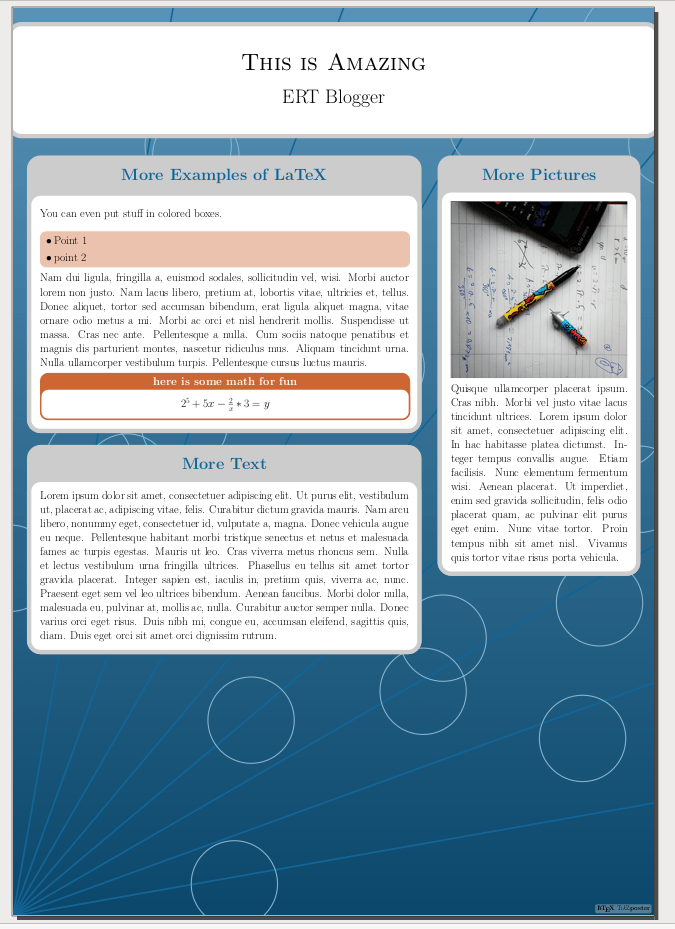Final Block

We will now put a block that runs along the bottom of the poster. Just a title with some text. This code must be placed above the \end{document} command.

\block{The End}{
\lipsum[10-11]
}Conclusion

Perhaps, you can see how cool and versatile LaTeX can be. You can make a poster for presentations that are rather beautiful and much more symmetrical than trying to draw boxes by hand using powerpoint.

# Lists in LaTeX VIDEO

Making list in LaTeX# Cross-Referencing with LaTeX

Cross-referencing allows you to refer to almost anything in your document automatically through the use of several LaTeX commands. This can become extremely valuable if you have to edit your document and things change. With whatever updates you make the cross-referencing is update automatically.There are many different ways to cross-reference in LaTeX but we will look at the following.

• Tables
• Items in a list
• Pages

When using cross-referencing you must compile the document twice in order for the numbers to show up. The first time you will only see question marks.

Tables

Below is an example of LaTeX referring to a table.

\documentclass{article}
\begin{document}
In Table~\ref{Example} we have an example of a table
\begin{table}[h]
\begin{center}
\begin{tabular}{ll}
\hline
Fruits&Vegetables\\
\hline
Mango&Lettuce\\
Papaya&Kale\\
\hline
\end{tabular}
\caption{Example} \label{Example}
\end{center}
\end{table}
\end{document}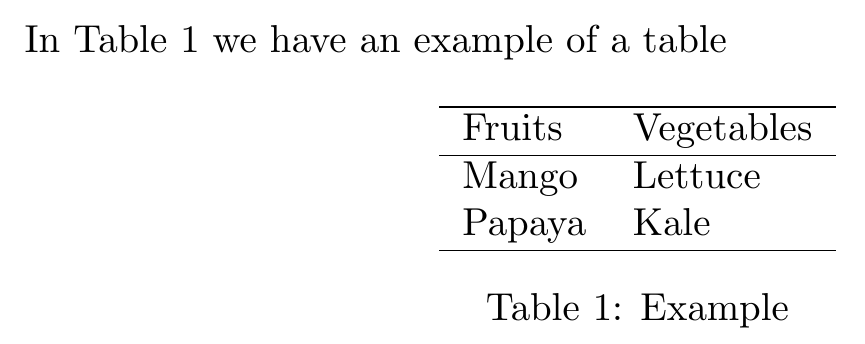How a table is created has been discussed previously, what is new here are two pieces of code.

• ~\ref{  }
• \label{ }

The “\label” declaration gives the table a label that can be used in the text. In the example, we labeled the table “Example”. The “~\ref” declaration is used in the text and you put the label name on the table inside the curly braces. If you look at the text we never use the number 1 in the text. LaTeX inserts this for us automatically.

The same process can be used to label images as well.

Item in List

Cross-referencing an item on a list is not that complex either. Below is an example.

\documentclass{article}
\begin{document}
Simple list
\begin{enumerate}
\item Mango
\item Papaya \label{fruit2}
\item Apple
\end{enumerate}
Number \ref{fruit2} is a common fruit in tropical countries.
\end{document}As you can see, you can label almost anything anywhere.

Referring to Pages

It is also possible to refer to pages. This can save a lot of time if you update a document and page numbers change. Below is the code and example.

\documentclass{article}
\begin{document}
Simple list \label{list}
\begin{enumerate}
\item Mango
\item Papaya \label{fruit2}
\item Apple
\end{enumerate}
Number \ref{fruit2} in the list on page~\pageref{list}
is a common fruit in tropical countries.
\end{document}We made a label right above our list and then we used the “~\pageref” declaration with the name of the label inside. This provides us with the page number automatically.

Conclusion

There are more complex ways to cross-reference. However, unless you are developing a really complex document they are not really necessary for most practical applications. The ideas presented here will work in most instances as they are.

# Insert Images into a LaTeX Document

We have all heard that a picture is worth a thousand words. Images help people to understand concretely what a writer is trying to communicate with text. In this post, we will look at how to include images inside documents prepared with LaTeX.Basic Example

One way to include an image is to use the “graphicx” package and to set the path for where the image is using the “\garphicspath” declaration in the preamble of a LaTeX document. Below is an example. Included in the example is the “babel” and “blindtext” packages to create some filler text.

\documentclass{article}
\usepackage[english]{babel}
\usepackage{blindtext}
\usepackage{graphicx}
\graphicspath{ {PUT THE PATH HERE} }
\begin{document}
\blindtext

\includegraphics[scale=.1]{1.jpg}

\blindtext
\end{document}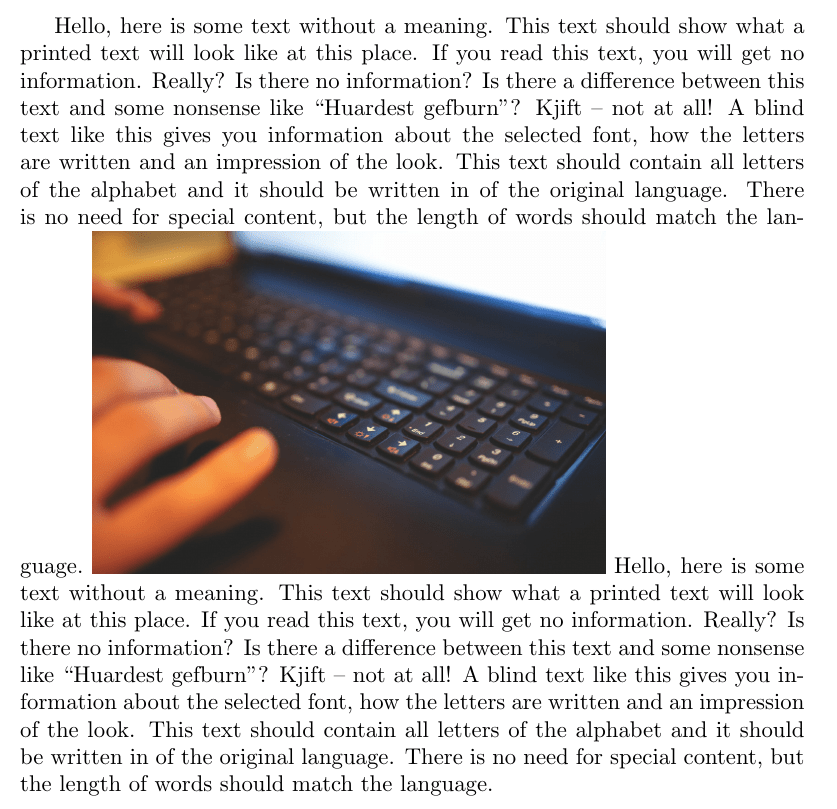Inside the actual document we use the following declaration

\includegraphics[scale=.1]{1.jpg}

“\includegraphics” is the declaration. The “scale” argument reduces the size of the image. The information in the curly braces is the name of the actual file. You can see that our print out is rather ugly and needs refinement.

One thing our picture needs is a caption that describes what it is. This can be done by first creating a figure environment, placing the “\includegraphics” declaration inside it, and using the “\caption” declration. Below is an example. We will also center the image for aesthetic reasons as well.

\documentclass{article}
\usepackage[english]{babel}
\usepackage{blindtext}
\usepackage{graphicx}
\graphicspath{ {PUT THE PATH HERE} }
\begin{document}
\blindtext

\begin{figure}
\centering
\includegraphics[scale=.1]{1.jpg}
\caption{Using Images in \LaTeX}
\end{figure}

\blindtext
\end{document}We created a figure environment added our image and type a caption. LaTeX automatically added “Figure 1” to the image. In addition,  you can see that the picture moved to the top of the page. This is because environments are able to float to the best position on a page as determined by calculations made by LaTeX.

If you want the image to appear in a particular place you can add the optional arguments h,t,b,p next to the “\begin{figure}” declaration. h =  here, t = top, b = bottom, and p = separate page.

To get rid of floating use the package called “capt-of”  and the declaration “\captionof{figure or table}{name here}}”. This will freeze the image in place so that it does not move all over the place as you add content to your document. Below is the same example but using the “capt-of” package.

\documentclass{article}
\usepackage[english]{babel}
\usepackage{blindtext}
\usepackage{graphicx}
\graphicspath{ {PUT PATH HERE} }
\usepackage{capt-of}
\begin{document}
\blindtext

\begin{center}
\includegraphics[scale=.1]{1.jpg}
\captionof{figure}{Using Images in \LaTeX}
\end{center}

\blindtext
\end{document}This is almost like our first example except now we have a caption. We did have to create a center environment but this type of environment does not float.

Wrapping Figures

The last example is wrapping text around a figure. For this, you need the “wrapfig” package and you need to create an environment with the “Wrapfigure” command. You also must indicate where the figure should be to the left (l),  center (c), or to the right (r). Lastly, you need to indicate the width of the figure. Below is the code followed by the results.

\documentclass{article}
\usepackage[english]{babel}
\usepackage{blindtext}
\usepackage{graphicx}
\usepackage{wrapfig}
\begin{document}
\blindtext

\begin{wrapfigure}{r}{7.8cm}
\includegraphics[scale=.1]{1.jpg}
\caption{Using Images in \LaTeX}
\end{wrapfigure}

\blindtext
\end{document}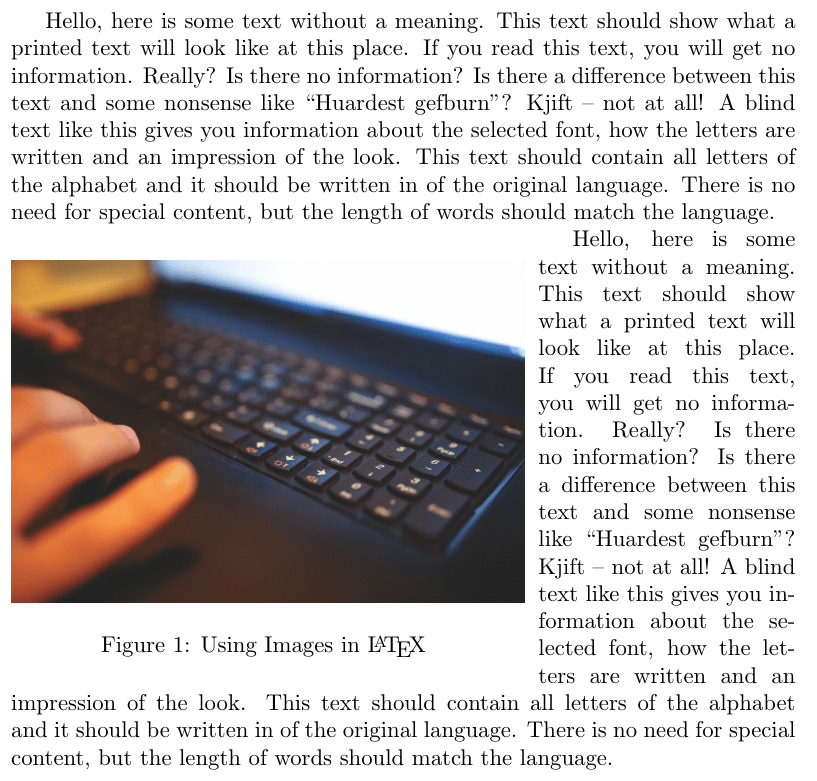In the example above, we moved the image to the left. For the width, you have to guess several times so that all of the text appears next to the figure rather than behind it.

Conclusion

This post provided several practical ways to include images in a LaTeX document. With this amount of control, you are able to make sophisticated documents that are consistently reproduced.

# Page Justification in LaTeX VIDEO

Page justification in LaTeX

# Making Commands with Options in LaTeX VIDEO

Making commands with options in LaTeX# Making Lists in LaTeX

Lists are frequently used in communication in order to provide information succinctly. Often, the rules of grammar can be suspended because of the need for the list to communicate information in an unadorned way. In this post, we will learn how to make lists in LaTeX.Basic List

For a simple list, you need to make an environment using the “itemize” declaration. Inside this environment, you must use the “\item” declaration for each bullet in the list. Below is the code and printout of a basic list in LaTeX.

\documentclass{article}
\begin{document}
Shopping list
\begin{itemize}
\item lettace
\item mango
\item toothpaste
\end{itemize}
\end{document}As you can see this is fairly simple. THere is no need for any packages to complete this. If you want a number list instead of creating a “itemize” environment you would create an “enumerate” environment as shown below.

\documentclass{article}
\begin{document}
Shopping list
\begin{enumerate}
\item lettace
\item mango
\item toothpaste
\end{enumerate}
\end{document}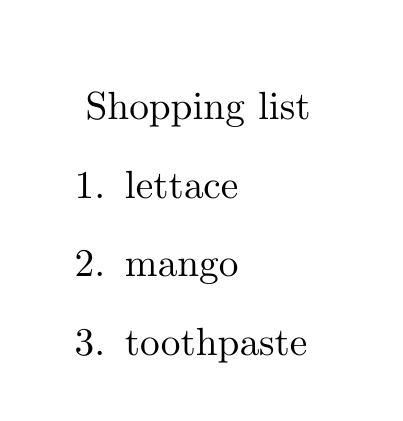Nested List

It is possible to have lists within lists. To do this, you simply create an environment within an environment. LaTeX will automatically change the bullet type for you to enhance readability. Below is an example.

\documentclass{article}
\begin{document}
Shopping list
\begin{itemize}
\item fruits
\begin{itemize}
\item lettace
\item mango
\end{itemize}
\item other
\begin{itemize}
\item toothpaste
\end{itemize}
\end{itemize}
\end{document}The example above has two levels in the list. LaTeX can go up to four levels.

Compact List

Generally, list by default in LaTeX are double-spaced. To reduce this you need to use the “paralist” package with either the “\compactitem” declaration and or the “\compactenum” declaration. Below is the same example but using the paralist features and also blending the use of bullets and numbers.

\documentclass{article}
\usepackage{paralist}
\begin{document}
Shopping list
\begin{compactitem}
\item fruits
\begin{compactenum}
\item lettace
\item mango
\end{compactenum}
\item other
\begin{compactenum}
\item toothpaste
\end{compactenum}
\end{compactitem}
\end{document}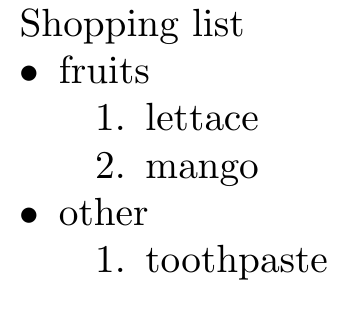Definition List

It is also possible to make a list of definition. This is useful for a glossary. In order to do this, you create a “description” environment. When you use the “\item” declaration you need to place the definition word in brackets. There are no packages needed for this. Below is the code.

\documentclass{article}
\usepackage{paralist}
\begin{document}
\begin{description}
\item[convoluted] complex and hard to understand
\item [obtuse] slow to understand
\end{description}
\end{document}Conclusion

This post provided insights into the use of lists in LaTeX.

# Making Commands in LaTeX VIDEO

Make commands in LaTeX# Line Breaks and Justification in LaTeX

In this post, we will look at line breaks and justification in LaTeX. These tools will provide a user with more nuanced command of their document.Paragraph Break

By leaving a space between paragraphs in your document LaTeX will start a new paragraph. Below is the code followed by the output.

\documentclass{article}
\begin{document}
Hello, here is some text without a meaning. This text should show what a printed text will look like at this place. If you read this text, you will get no information.

Really?

Is there no information?

Is there a difference between this text and some nonsense like “Huardest gefburn”?

Kjift – not at all!

A blind text like this gives you information about the selected font.
\end{document}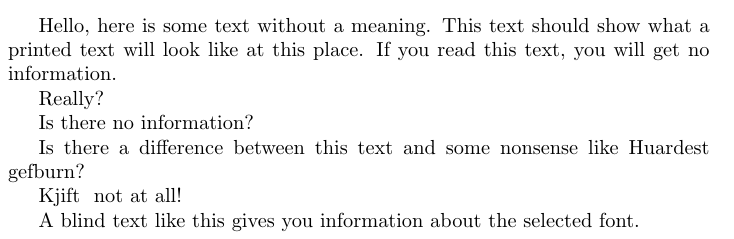Notice how each paragraph is indented. This is the default setting in LaTex. To remove indentation you need to use the “\noindet” declaration as shown below.

\documentclass{article}
\begin{document}
Hello, here is some text without a meaning. This text should show what a printed text will look like at this place. If you read this text, you will get no information.

\noindent

Really?
\noindent

Is there no information?
\noindent

Is there a difference between this text and some nonsense like “Huardest gefburn”?
\noindent

Kjift – not at all!
\noindent

A blind text like this gives you information about the selected font.
\end{document}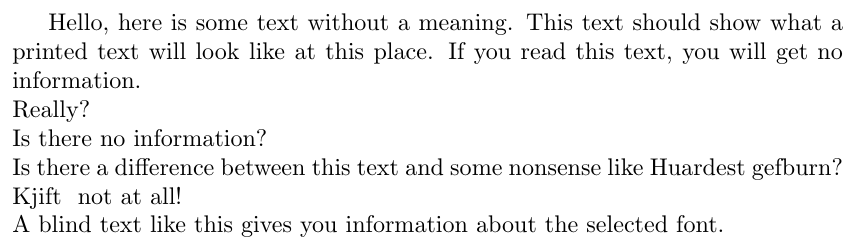In this example, only the first paragraph is indented.

A simpler way to do this is with the short command line break \\. Below is what it looks like

\documentclass{article}
\begin{document}
Hello, here is some text without a meaning. This text should show what a printed text will look like at this place. If you read this text, you will get no information.\\
Really?\\
Is there no information? \\
Is there a difference between this text and some nonsense like “Huardest gefburn”? \\
Kjift – not at all! \\
A blind text like this gives you information about the selected font.
\end{document}You can see that both “\noindent” and the short command \\ get the same results. However, the latter is probably more efficient and perhaps easier to read.

Justification

There are also ways to remove the default setting for justification. The three declaration are “\raggedright”, “\raggedleft”, and “\centering”. The “\raggedright” declaration makes the right side of the page ragged while the left side of the page is justified as shown below.

\documentclass{article}
\usepackage[english]{babel}
\usepackage{blindtext}
\begin{document}
{\raggedright
\Blindtext}
\end{document}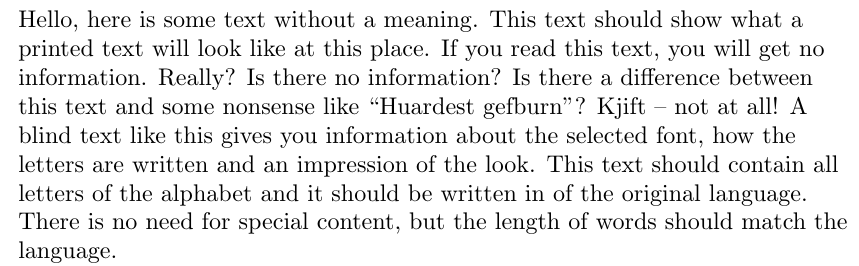You can clearly see how the right side is truly ragged. The other packages in the code create the demo paragraph automatically for us.

The “\raggedleft” declaration does the opposite. See below

\documentclass{article}
\usepackage[english]{babel}
\usepackage{blindtext}
\begin{document}
{\raggedleft
\Blindtext}
\end{document}I think we already know what centering does.

\documentclass{article}
\usepackage[english]{babel}
\usepackage{blindtext}
\begin{document}
{\centering
\Blindtext}
\end{document}Conclusion

This post provided a demonstration of line breaks and justification in LaTeX.

# Modifying Text in LaTeX VIDEO

Basic modifications of text in LaTeX

# Modifying Text and Creating Commands in LaTeX

In this post, we are going to explore to separate features available in LaTeX. These two features are modifying the text size and creating custom commands.

Modifying TextYou can change the size and shape of text using many different declarations/environments in LaTeX. Declarations and environments serve the same purpose the difference is in the readability of the code. In the example below, we use an environment to make the text bigger than normal. The code is first followed by the example

\documentclass{article}
\usepackage[english]{babel}
\usepackage{blindtext}
\begin{document}
\begin{huge}
\blindtext
\end{huge}
\end{document}Here is what we did.

1. We create a document with the class of article
2. We used the “babel” and “blindtext” packages to create some filler text.
3. Next, we began the document
4. We create the environment “huge” for enlarging the text.
5. We used the declaration  “\blindtext” to create the paragraph
6. We closed the “huge” environment with the “end” declaration
7. We end the document

If you ran this code you will notice the size of the text is larger than normal. Of course, you can bold and do many more complex things to the text simultaneously. Below is the same example but with the text bold and in italics

\documentclass{article}
\usepackage[english]{babel}
\usepackage{blindtext}
\begin{document}
\begin{huge}
\bfseries
\textit
\blindtext
\end{huge}
\end{document}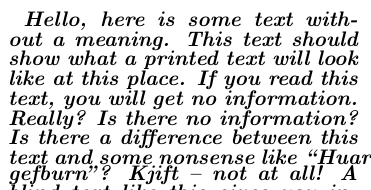The code is mostly the same with the addition of “\bfseries” for bold and  “\texit” for italics.

Making Commands

It is also possible to make custom commands in LaTeX. This can save a lot of time for repetitive practices. In the example below, we create a command to automatically print the name of this blog’s web address.

\documentclass{article}
\newcommand{\ert}{\bfseries{educationalresearchtechniques}}
\begin{document}
The coolest blog on the web is \ert
\end{document}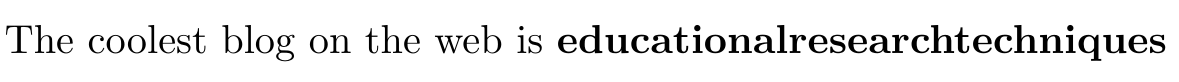In the code, we use the declaration “\newcommand” in the preamble. This declaration had the command “\ert” which is the shorthand for the code to the right which is “\bfseries{educationalresearchtechniques}. This code tells LaTeX to bold the contents inside the brackets.

The next step was to begin the document. Notice how we used the “\ert” declaration and the entire word educationalresearchtechniques was printed in bold in the actual pdf.

It is also possible to make commands that format text. Below is an example.

\documentclass{article}
\newcommand{\mod}{\textbf{\textit{#1}}}
\begin{document}
The is an example of modified \mod{text}
\end{document}What is new is in line 2. Here we use the “\newcommand” declaration again but this time we create a command call “\mode” and give it an argument of 1 (see ) this is more important when you have more than one argument. Next, we put in curly brackets what we want to be done to the text. Here we want the text to be bold “\textbf” and in italics “\textit”. Lastly, we set the definition {#1}. Definition works with arguments in that argument 1 uses definition 1, argument 2 uses definition 2, etc.  Having more than one argument and definition can be confusing for beginners so this will not be explored for now.

Conclusion

This post provided assistance in understanding LaTeX’s font size capabilities as well as ways to make new commands.

# Setup a Document in LaTeX VIDEO

Here is a basic example of setting up a document in LaTeX.

# Intro LaTeX VIDEO

Introducing LateX# Basics of LateX

In this post, we will explore more concepts about Latex the typesetting language.Optional Commands

Optional commands appear in brackets [   ]  when you are using Latex. In the example below, we will set the font size to 20pt in the preamble of the document. The code is as follows.

\documentclass[4paper,12pt]{article} \begin{document}
Behold the power of \LaTeX
\end{document}

Here is what it looks like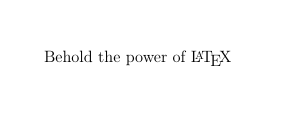Inside the brackets, we set the paper size to A4 and the font size to 12pt. Many if not most commands have optional commands that can be used to customize the behavior of the document.

Like most coding languages Latex allows you to make comments. To do this you need to place a % sign in front of your comment. As shown below

\documentclass[4paper,12pt]{article} \begin{document}
Behold the power of \LaTeX
%This will not print
\end{document}Everything after the % did not print. To stop this action simply press enter to move to the next line and you can continue with your document.

Fun with Fonts

There are many different ways to set the fonts. Generally, you can use the \text**{  } code. Where the asterisks are is where you can specify the behavior you want of the text. Below is a simple example of the use of several different formats to the font.

\documentclass[4paper,12pt]{article}
\begin{document}
You can \textit{italicized}
Text can be \textsl{slanted}
Off course, you can \textbf{bold} text
You can also make text in \textsc{small caps}
It is also possible to use several commands at the same \textit{\textbf{time}}
Behold the power of \LaTeX
\end{document}Notice how you put the command in front of the word that you want to format. This might seem cumbersome. However, once you get comfortable with this it is much faster to format documents then the point and click style of Word.

Environments

If you want a certain effect to last for awhile you can use an environment. An environment is a space you declare in your document in which a center behavior takes place. Generally, environments are used to improve the readability of your code. Below is an example.

\documentclass[4paper,12pt]{article}
\begin{document}
\begin{bfseries}
Everything is bold here
\end{bfseries}
\begin{itshape}
Everything is bold here
\end{itshape}
Behold the power of \LaTeX
\end{document}An environment always begins with the \begin command and ends with the \end command. In the curly braces, you type whatever is required for your formatting goals. There are scores of commands you can place inside the curly braces.

Conclusion

There is so much more to learn but this is just a beginning. One of the main benefits of learning Latex is the fixed nature of the formatting and the speed at which you can produce content once you are familiar with how to use this language.# Intro to LaTeX

History

LaTex is an open-sourced typesetting document developed about 30 years ago by Leslie Lamport and based on the Tex typesetting of Donald Knuth. It is commonly used in the domains of physics and math for producing mathematical equations and other technical documents. Below is a simple example of an equation developed using LaTexLaTex is a document markup language, which means that you indicate the commands and then it is processed to produce the desired effect. This is in contrast to Microsoft Word which utilizes a WYSIWYG (What you see is what you get) approach.

Benefits

Using LaTex provides several benefits. Cross-referencing is easily accomplish especially with the help of BibTex. It is also multi-lingual and able to make glossaries, indexes, and figures/tables with ease. In addition, LaTex is highly portable and opening a file on any computer is not a problem. Sometimes moving to another computer using Microsoft Word can cause issues with formatting.

Another benefit is psychology, using LaTex allows the author to focus on content and not appearance when writing. It is easy to get distracted when using Word to try and make something work through the point and click mechanism we are so used to when writing.

Cons

It takes extensive time to use LaTex. It looks similar to coding which is intimidating for many. However, once a certain mastery is achieved. Producing documents can be faster as everything is text-based and not point click based using a mouse.

Using LaTex

To use LaTex you need to install TexLive and TexWorks. TextLive is a LaTex distribution and TexWorks is one of many LaTex editors. The editor allows you to manipulate the LaTex code that you generate.

Once you have installed both programs you can type the following into TexWorks. Make sure the typeset is set to pdfLaTex. This allows the output to be a pdf file.

\documentclass{article}
\begin{document}
This is an example of what LaTex does
\end{document}What happened is as follows

1. We entered the command \documentclass{article}. All commands begin with a slash followed by the name. The curly braces are required arguments. In this case, we are using the article template which is one of many templates available in LaTex.
2. The next command is \begin and this command indicates the beginning of the actual text of the document. Everything above the \begin command is part of what we call the preamble.
3. Next is the actual text that we want to appear in the pdf.
4. Lastly, we have the \end command which tells LaTex that the document is finished. Everything between \begin and \end command is part of the environment.

Conclusion

There is so much more that can be accomplished with this typesetting software. The possibilities will be explored in the near future.